# ISEE Lower Level Quantitative : How to find the common part with a Venn Diagram

## Example Questions

### Example Question #1 : How To Find The Common Part With A Venn Diagram

Students were asked if they prefer TV or radio. The following Venn Diagram depicts the number of students who said TV, radio, or both. How many students like both TV and radio?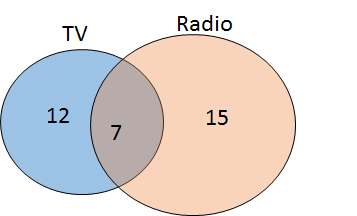12

22

15

7

7

Explanation:

The blue circle of the Venn diagram depicts the number of students who prefer TV, the orange circle depicts the number of students who prefer radio, and the region of overlap indicates the number of students who like both. Therefore, 7 students like both TV and radio.

### Example Question #1 : How To Find The Common Part With A Venn DiagramAracely posted a survey question using one of her social network accounts. She grouped the results into three categories. The response results are represented by the Venn diagram shown above.

Group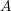represents the respondents that answered "yes" to the survey question. Group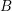represents the respondents that answered "no" to the survey question. And, the overlapping part of the diagram represents the respondents that answered "maybe" to the survey question.

What percentage of the respondents answered "maybe" to Aracely's survey question?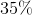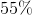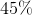Explanation:

The overlapping portion of the Venn diagram represents the percentage of respondents that answered "maybe" to Aracely's survey question.

Since the diagram shows that exactly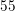percent of the respondents answered "yes" or "no" to the question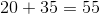.

The solution is the total percent population take away the percent that answered "yes" or "no":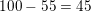### Example Question #2 : How To Find The Common Part With A Venn Diagram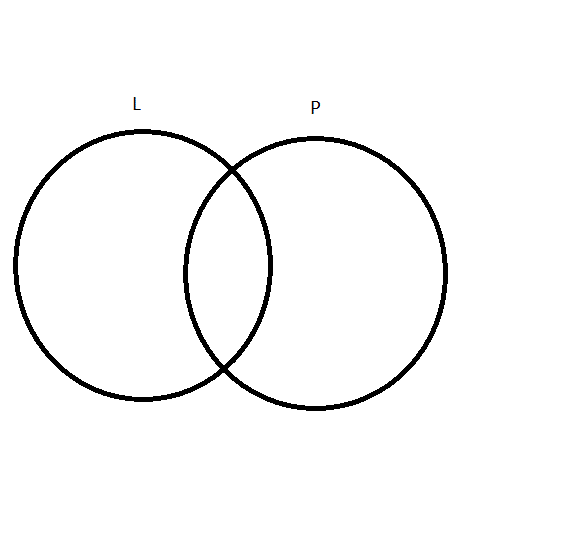The Venn diagram shown above has three categories that represent information about the Wildcats varsity baseball team.

Category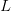represents the number of players on the team that are left-handed.
Category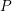represents the numebr of players on the team that are pitchers.
And, the overlapping portion of the Venn diagram represents the number of players that are left-handed pitchers.

Given that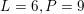and that there are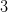players in the overlapping region of the diagram.

What fraction of the players are left-handed pitchers?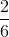Explanation:

Since there areleft-handed pitchers and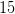total players that are either left-handed, pitchers or both the solution is: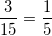### Example Question #3 : How To Find The Common Part With A Venn Diagram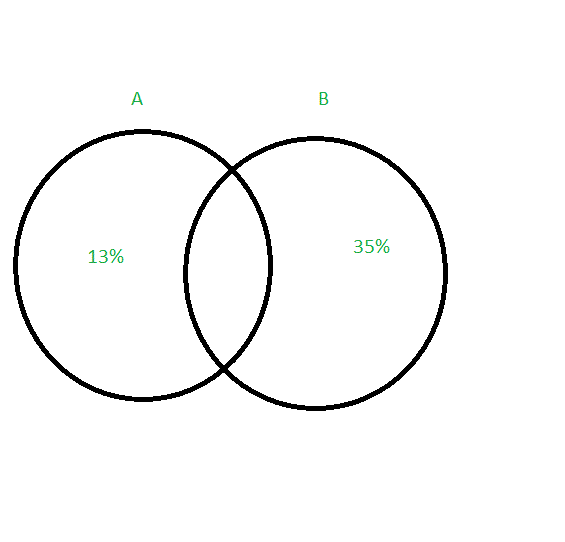The Venn diagram above represents the results from a recent survey given to middle school students. Categoryrepresents the amount of students that only like pizza. Categoryrepresents the amount of students that only chicken nuggets.

What percentage of students like both chicken nuggets and pizza?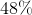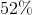Explanation:

Since we are asked to find the percent of students that like both chicken nuggets and pizza we need to first add the percentages together.

Thus we get,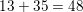.

The amount of students that like both pizza and chicken nuggets is going to be all of the students minus those that like only chicken nuggets and only pizza.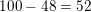.

### Example Question #4 : How To Find The Common Part With A Venn DiagramThe Venn diagram above represents the results from a recent survey given to middle school students. Categoryrepresents the amount of students that only like pizza. Categoryrepresents the amount of students that only chicken nuggets.

What percentage of students like both chicken nuggets and pizza?Explanation:

Since we are asked to find the percent of students that like both chicken nuggets and pizza we need to first add the percentages together.

Thus we get,.

The amount of students that like both pizza and chicken nuggets is going to be all of the students minus those that like only chicken nuggets and only pizza..